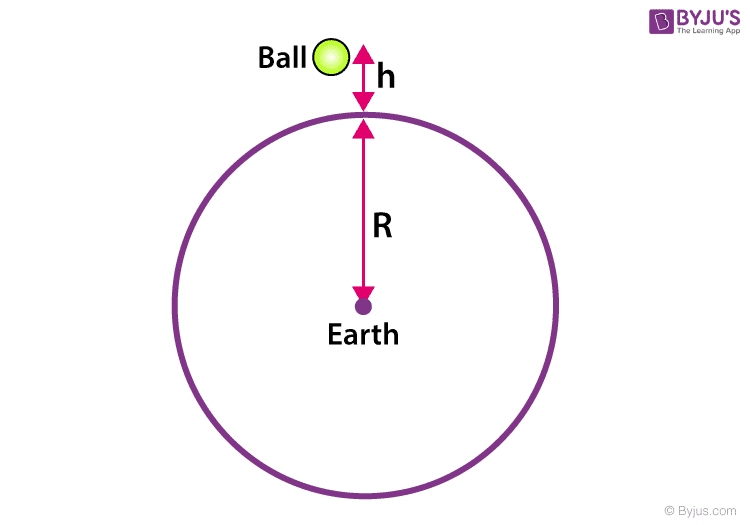# Visualizing Motion during a Free Fall

When we drop the ball, let’s say from the top floor of a building, then till it reaches the ground, the ball is said to be in a free-fall motion.

## What Is Free Fall?

Freefall is defined as a situation when a body is moving only under the influence of the earth’s gravity. Since external force is acting on the ball, the motion will be accelerated. This free-fall acceleration is also known as acceleration due to gravity. Let us find the value acceleration due to gravity during free fall. To find this, we take one assumption that the height from which the ball is dropped is very small as compared to the radius of the earth.Force acting during free fall = Force of gravitation between earth and ball

$$\begin{array}{l}F = \frac{GMm}{(R+h)^2}\end{array}$$

We have assumed

$$\begin{array}{l}R + h ~ R\end{array}$$
$$\begin{array}{l}F = \frac{GMm}{R^2}———-(1) \end{array}$$
According to Newton’s second law,
$$\begin{array}{l}F = ma\end{array}$$

Freefall acceleration or acceleration due to gravity is represented by ‘g’.

$$\begin{array}{l}F = mg———-(2)\end{array}$$

Using equations (1) and (2),

$$\begin{array}{l}mg = \frac{GMm}{R^2}\end{array}$$
$$\begin{array}{l}g = \frac{GM}{R^2}———-(3)\end{array}$$

Where M = mass of the earth and R = radius of the earth

Now the next that comes to mind that we have already seen is ‘G’, i.e. Universal Gravitational Constant. Its value remains the same everywhere. But is it true for g? From equation (3), we can see that g depends on the dimension of the body, i.e. mass and radius. Hence, it will not be the same everywhere. Also, as the acceleration remains constant during free-fall motion, so we can use equations of motion. We just have to replace the value of acceleration in all the equations with g.

For example, v = u + gt## Frequently Asked Questions – FAQs

Q1

### What is meant by freefall?

Freefall can be defined as a scenario when an object is moving only under the effect of the Earth’s gravitational pull. As external force is exerted on the object, the motion will be constantly accelerated. This is known as freefall acceleration.

Q2

### What is meant by weight?

Weight is the measure of the force of gravity acting on a body.

The formula for weight is given by:

w = mg

Q3

### What is weightlessness?

Weightlessness is a condition of free-fall in which the gravity is cancelled by the inertial force arising from orbital flight or other gravity-negating situations. It is experienced due to the absence of the feeling of weight. It happens in the lack of any contact forces acting on bodies (human body).

Q4

### What is inertia?

Inertia is a scalar quantity which is the measure of the resistance exerted by an object to any change in velocity.

Q5

### What is acceleration?

Acceleration is defined as the rate of change of motion of a body. In other words, the measure of the rate of change in its speed along with direction with respect to time is called acceleration.

Q6

### What is linear acceleration?

The motion of the object can be linear or circular. Thus, the acceleration involved in linear motion is called linear acceleration.

#### The full summary of the chapter Gravitation Class 9#### The important questions in the chapter Gravitation Class 9Learn science the right way! Join BYJU’S. Join us and fall in love with learning.

Test Your Knowledge On Free Fall!